# Bullets stuck in a log and speed it up

## Homework Statement

A cannon shoots bullets of mass m0 and velocity v0 at a massive log of wood of mass 100m0. the interval between the bullets is t0. the surface is smooth. the bullets are stuck in the wood. the penetration time of the bullets is very short, relative to t0.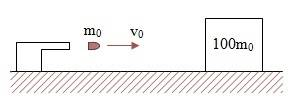1) What's the log's velocity after the first bullet.
2)What's the log's velocity after N bullets.
3) Express the number of the bullets in the log as a function of it's velocity.
4) How much heat energy was created in the log after N bullets.
5) How much time elapsed between the N-1 bullet hit and the Nth bullet hit.
6) How much time elapsed between the first bullet's hit and the Nth bullet's hit.
7) When does the log's velocity equal 0.5v0.

## Homework Equations

Conservation of momentum: ##\sum m_i v_i=\sum m_i v'_i##
Kinetic energy: ##\frac{1}{2}mv^2##

## The Attempt at a Solution

After the first bullet:
$$m_0v_0=(100+1)m_0v\;\rightarrow\; v=\frac{1}{101}v_0$$
The total momentum after N bullets is ##P=Nm_0v_0## . the velocity must cancel with a part of this momentum to give back the same P, so:
$$Nm_0v_0=(100+N)m_0\frac{Nv_0}{100+N}m_0\;\rightarrow\; v_N=\frac{Nv_0}{100+N}$$
The number of bullets as a function of the velocity:
$$\frac{v_0}{v_N}=\frac{100}{N}+1\quad\rightarrow\;N=\frac{100v_N}{v_0-v_N}$$
The velocity of the log after N-1 bullets is ##v_{N-1}=\frac{(N-1)v_0}{99+N}## (derived from the vN formula). so the energy loss between the (N-1)th and the Nth bullets is:
$$\Delta E=\frac{1}{2}\left[ m_0v_0^2+(99+N)m_0\frac{(N-1)^2v^2_0}{(99+N)^2}-(100+N)m_0\frac{N^2v^2_0}{(100+N)^2} \right]=\frac{m_0v^2_0}{2}\left[ 1+\frac{(N-1)^2}{99+N}-\frac{N^2}{100+N} \right]$$
The total energy loss from the first bullet to the Nth is the sum of these ΔE's, which is complicated. i don't think this is the best method.
The distance the log travells between the (N-1) and the Nth bullets is:
$$\Delta s=v_{N-1}\cdot t_0=\frac{(N-1)v_0t_0}{99+N}$$
The time between the (N-1)th and the Nth bullets is, therefore:
$$\Delta s=v_0\cdot t\quad\rightarrow\; t_{(N-1)\rightarrow N}=\frac{\Delta s}{v_0}=\left( 1+\frac{N}{99+N} \right)t_0$$
The time elapsed between the first and the Nth bullets is the sum of the above time, which is complex and isn't expected to be found in this way.
The velocity of 0.5v0 will be reached after:
$$\frac{Nv_0}{100+N}\leqslant \frac{v_0}{2}\quad\rightarrow\; N\leqslant 100$$

ehild
Homework Helper

## Homework Statement

4) How much heat energy was created in the log after N bullets.

The velocity of the log after N-1 bullets is ##v_{N-1}=\frac{(N-1)v_0}{99+N}## (derived from the vN formula). so the energy loss between the (N-1)th and the Nth bullets is:
$$\Delta E=\frac{1}{2}\left[ m_0v_0^2+(99+N)m_0\frac{(N-1)^2v^2_0}{(99+N)^2}-(100+N)m_0\frac{N^2v^2_0}{(100+N)^2} \right]=\frac{m_0v^2_0}{2}\left[ 1+\frac{(N-1)^2}{99+N}-\frac{N^2}{100+N} \right]$$
The total energy loss from the first bullet to the Nth is the sum of these ΔE's, which is complicated. i don't think this is the best method.
Why do you do it so complicated? What is the input of KE during N shots? What is the resulting KE?
If you want to add the loss of KE in every step, it will be (KE(bullet) - KE(1)) +( KE(bullett+ KE(1)-KE(2)) +...

What is the input of KE during N shots? What is the resulting KE?
$$\Delta EK=\frac{1}{2}Nm_0v_0^2-\frac{1}{2}(100+N)\frac{N^2v_0^2}{(100+N)^2}=\frac{50Nm_0v_0^2}{100+N}$$
But how to find the time from the first bullet hit to the Nth hit? i don't see a method other than adding the individual times, which depend each on a different velocity

But how to find the time from the first bullet hit to the Nth hit? i don't see a method other than adding the individual times, which depend each on a different velocity

SammyS
Staff Emeritus
Homework Helper
Gold Member

## Homework Statement

A cannon shoots bullets of mass m0 and velocity v0 at a massive log of wood of mass 100m0. the interval between the bullets is t0. the surface is smooth. the bullets are stuck in the wood. the penetration time of the bullets is very short, relative to t0.
View attachment 100398
1) What's the log's velocity after the first bullet.
2)What's the log's velocity after N bullets.
3) Express the number of the bullets in the log as a function of it's velocity.
4) How much heat energy was created in the log after N bullets.
5) How much time elapsed between the N-1 bullet hit and the Nth bullet hit.
6) How much time elapsed between the first bullet's hit and the Nth bullet's hit.
7) When does the log's velocity equal 0.5v0.
What is the initial velocity of the log ?

ehild
Homework Helper
5) How much time elapsed between the N-1 bullet hit and the Nth bullet hit.

The distance the log travels between the (N-1) and the Nth bullets is:

$$\Delta s=v_{N-1}\cdot t_0=\frac{(N-1)v_0t_0}{99+N}$$
The time between two subsequent bullet hits is not to. to is the time between the shots. The bullet hits the log between the shots, and before the (N-1)-th hit the log travels with VN-2 velocity, after the hit it travels with VN-1. To get the distance the log travels between the hits is quite complicated.
Edit: I think I have found the solution. The results are quite simple.

Last edited:
ehild
Homework Helper
What is the initial velocity of the log ?
It is assumed zero.

ehild
Homework Helper
Assume the N-1-th shot happens at tsN-1=0. At that instant, the log is at xs(N-1)=D and its speed is V(N-2). What time is the impact?
What is the position xi(N-1) of the log at the impact?
Where is log at instant of the N-th shot? (What is xs(N) at ts(N)=t0?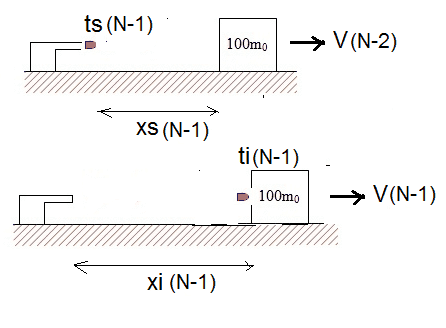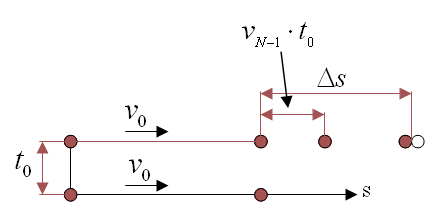$$\Delta s=v_0\cdot t=v_{N-1}\cdot t_0+v_{N-1}\cdot t~~~\rightarrow~~~t_{(N-1)\rightarrow N}=\frac{N-1}{100}t_0$$
The total time for N hits:
$$\sum^N_1 t_0=\frac{(N-1)}{100}=\frac{1}{100}\left[ \sum^N_1-N \right]=\frac{1}{100}\left[ \frac{N(1+N)}{2} \right]=\frac{N(1+N)}{50}t_0$$

ehild
Homework Helper
View attachment 100515
$$\Delta s=v_0\cdot t=v_{N-1}\cdot t_0+v_{N-1}\cdot t~~~\rightarrow~~~t_{(N-1)\rightarrow N}=\frac{N-1}{100}t_0$$
The total time for N hits:
$$\sum^N_1 t_0=\frac{(N-1)}{100}=\frac{1}{100}\left[ \sum^N_1-N \right]=\frac{1}{100}\left[ \frac{N(1+N)}{2} \right]=\frac{N(1+N)}{50}t_0$$
And what do you mean on total time of hits?
What is your answer to question "How much time elapsed between the N-1 bullet hit and the Nth bullet hit"?
Your last line is totally wrong.

Last edited:
Sorry, missed some things on the last line.
The situation is like of a police car starting fast to catch a thief's car, which starts further in front but drives slower. the cars will meet at distance Δs.
The starting point of the police car is where bullet (N-1) hit the log. if the log didn't move, the next bullet, the N-th, would hit at the same place, which is the start for the police car.
##t_{(N-1)\rightarrow N}## is the time elapsed between the (N-1) hit and the N-th:
$$\Delta s=v_0\cdot t_{(N-1)\rightarrow N}=v_{N-1}\cdot t_0+v_{N-1}\cdot t_{(N-1)\rightarrow N}~~~\rightarrow~~~t_{(N-1)\rightarrow N}=\frac{N-1}{100}t_0$$
The last line displays the time elapsed from the first to the N-th hit:
$$\sum^N_1 \frac{N-1}{100}t_0=\frac{t_0}{100}\left[ \sum^N_1 N-N \right]=\frac{t_0}{100}\left[ \frac{N(1+N)}{2}-N \right]=\frac{N(N-1)}{50}t_0$$

ehild
Homework Helper
Sorry, missed some things on the last line.
The situation is like of a police car starting fast to catch a thief's car, which starts further in front but drives slower. the cars will meet at distance Δs.
The starting point of the police car is where bullet (N-1) hit the log. if the log didn't move, the next bullet, the N-th, would hit at the same place, which is the start for the police car.
##t_{(N-1)\rightarrow N}## is the time elapsed between the (N-1) hit and the N-th:
$$\Delta s=v_0\cdot t_{(N-1)\rightarrow N}=v_{N-1}\cdot t_0+v_{N-1}\cdot t_{(N-1)\rightarrow N}~~~\rightarrow~~~t_{(N-1)\rightarrow N}=\frac{N-1}{100}t_0$$
The shots follow each other in t0 intervals. The elapsed time between two hits can not be shorter than t0. From your formula, the time between the first and second hit is 0.01 t0.
You determined the time between the N-th shot and impact. To get the time between the impacts, add t0.

Last edited:
I got this for the 5th question.

Where ##T_2## is the time elapsed between the N-1 bullet hit and the Nth bullet hit.
## T_2 = \frac{T_o (N+99)}{100}##

When I looked at your solution, It seems you have thought of the same idea as mine. (Which I think is correct, waiting for confirmation)
This should be the correct formula
$$\Delta s=v_0\cdot t_{(N-1)\rightarrow N}=v_{o}\cdot t_0+v_{N-1}\cdot t_{(N-1)\rightarrow N}$$

ehild
Homework Helper
I got this for the 5th question.

Where ##T_2## is the time elapsed between the N-1 bullet hit and the Nth bullet hit.
## T_2 = \frac{T_o (N+99)}{100}##
When I looked at your solution, It seems you have thought of the same idea as mine. (Which I think is correct, waiting for confirmation)
This should be the correct formula
$$\Delta s=v_0\cdot t_{(N-1)\rightarrow N}=v_{o}\cdot t_0+v_{N-1}\cdot t_{(N-1)\rightarrow N}$$
Yes, that is the correct formula.

Last edited:
Yes, that is the correct formula.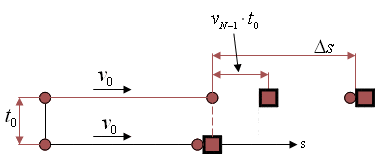It's not, the log has moved a distance, not the bullet, so the term ##v_0\cdot t_0## on the right isn't correct.
My formula on post #11 is correct:
$$\Delta s=v_0\cdot t_{(N-1)\rightarrow N}=v_{N-1}\cdot t_0+v_{N-1}\cdot t_{(N-1)\rightarrow N}$$
But if i extract ##t_{(N-1)\rightarrow N}## from the "wrong" formula i get the right answer:
$$t_{(N-1)\rightarrow N}=\frac{99+N}{100}$$
So why, despite of it, is it correct?

ehild
Homework Helper
View attachment 100558
It's not, the log has moved a distance, not the bullet, so the term ##v_0\cdot t_0## on the right isn't correct.
My formula on post #11 is correct:
$$\Delta s=v_0\cdot t_{(N-1)\rightarrow N}=v_{N-1}\cdot t_0+v_{N-1}\cdot t_{(N-1)\rightarrow N}$$
But if i extract ##t_{(N-1)\rightarrow N}## from the "wrong" formula i get the right answer:
$$t_{(N-1)\rightarrow N}=\frac{99+N}{100}$$
So why, despite of it, is it correct?
You do not get the correct result from your equation. In post #12 you got (N-1)/100 *to. It followed from your equation.

You do not get the correct result from your equation. In post #12 you got (N-1)/100
Yes but if i add t0 it's correct.
But the main thing for me is that i don't understand the reasoning for the term ##v_0t_0##.
I am beginning to understand but not yet. if i use:
$$\Delta s=v_0\cdot t_{(N-1)\rightarrow N}=v_{o}\cdot t_0+v_{N-1}\cdot t_{(N-1)\rightarrow N}$$
$$\Delta s=v_0\cdot t_{(N-1)\rightarrow N}=v_{N-1}\cdot t_0+v_{N-1}\cdot t_{(N-1)\rightarrow N}$$

Last edited:
ehild
Homework Helper
Yes but if i add t0 it's correct.
But the main thing for me is that i don't understand the reasoning for the term ##v_0t_0##.
I am beginning to understand but not yet. if i use:
$$\Delta s=v_0\cdot t_{(N-1)\rightarrow N}=v_{o}\cdot t_0+v_{N-1}\cdot t_{(N-1)\rightarrow N}$$
The time you got first was between the N-th shot and the N-th impact.
You said that you counted distance from the place of the N-1-th impact. That is a fixed place, and the next shot arrives there to time later. That is why you need to add to to your time.

You said that you counted distance from the place of the N-1-th impact. That is a fixed place, and the next shot arrives there to time later. That is why you need to add to to your time.
Exactly, that's what i understand, but what's the physical reasoning for the member ##v_0t_0## instead of ##v_{(N-1)\rightarrow N}t_0##?
Mathematically it adds my missing t0, but i don't see the physics of it. is it just a mathematical trick? if so, how did you do this, how did you know in advance what to do (if it's merely a mathematical trick, with no physical meaning)?
Edit: i think i am beginning to understand, you translated time to distance, there is no complete physical meaning. you need to get t0 plus something, so for the first t0 you need a distance of ##v_0t_0##, is that correct?

ehild
Homework Helper
The N-th bullet arrives to the place of the N-th impact t0 time later than the previous impact was. From the place of N-th impact, the N-th bullet moves with v0. The log is at distance VN-1to already. So the time the N-th bullet reaches the log is ##\frac {t_0 V_{N-1}}{v_0-V_{N-1}}##. You have to add t0 to get the time between the impacts. It is ##\frac {t_0 V_{0}}{v_0-V_{N-1}}##

The N-th bullet arrives to the place of the N-th impact t0 time later than the previous impact was
You must mean that the N-th bullet arrives to the place of the (N-1)-th impact, right?
Any way, you must mean point A in the drawing, right?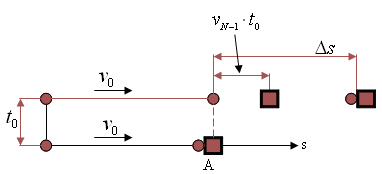##\frac {t_0 V_{N-1}}{v_0-V_{N-1}}## must be from the point of view of an observer riding on the log, and the observer sees the distance ##t_0V_{N-1}## closing with the relative velocity ##v_0-V_{N-1}##.
All this is correct but how to get from here to:
$$\Delta s=v_0\cdot t_{(N-1)\rightarrow N}=v_{o}\cdot t_0+v_{N-1}\cdot t_{(N-1)\rightarrow N}$$
I don't know.

You must mean that the N-th bullet arrives to the place of the (N-1)-th impact, right?
Any way, you must mean point A in the drawing, right?
View attachment 100573
##\frac {t_0 V_{N-1}}{v_0-V_{N-1}}## must be from the point of view of an observer riding on the log, and the observer sees the distance ##t_0V_{N-1}## closing with the relative velocity ##v_0-V_{N-1}##.
All this is correct but how to get from here to:
$$\Delta s=v_0\cdot t_{(N-1)\rightarrow N}=v_{o}\cdot t_0+v_{N-1}\cdot t_{(N-1)\rightarrow N}$$
I don't know.
This is how I see it.

Imagine a pretty accurate machine that shoots bullets, Now at an instance, the bullet number #1 for example was shot. After ##T_o## The bullet number #2 was shot. So the bullet number #1 moved with velocity ##V_o## for a time of ##T_o## before the bullet number #2 was shot. So ideally the distance between them will be ## d = V_o t_o##

Now does the distance change? Nope.... The velocities are equal to each other so it is fixed along the distance that they move.

For our N bullet and N-1 bullet. Skip the whole scene of N-1 catching the log. Once the N-1 bullet hits the log, What is the distance between the log and N's bullet? Remember what we said above.

What is the velocity of the log now? What is the velocity of the bullet? Calculate the time needed for the bullet to hit the log.

It is just the same as the cop and the thief examples. They have initial distance and the cop's car have a fast velocity compared to the velocity of the thief. (Poor Thief)

What you were doing wrong is when you calculate the distance you were using ## V_{N-1}## not ##V_o##

P.S: This assumes that the bullet penetrates the log in a very short time because the result would be difficult if it is not
the interval between the bullets is t0. the surface is smooth. the bullets are stuck in the wood. the penetration time of the bullets is very short, relative to t0.

•ehild
ehild
Homework Helper
You must mean that the N-th bullet arrives to the place of the (N-1)-th impact, right?
Any way, you must mean point A in the drawing, right?
Yes. And the Nth bullet catches the log after covering distance Δs
##\Delta s=v_0\cdot {\Delta t }=v_{N-1}\cdot t_0+v_{N-1}\cdot \Delta t##
from what you get the catch time
$$\Delta t=\frac{V_{N-1} \cdot t_0}{v_0-V_{N-1}}$$
The Nth bullet arrived at the place of the N-1th impact t0 time later than the N-1th bullet. So the time between the two impacts is
$$t_{(N-1)\rightarrow N}=t_0+\Delta t= t_0+\frac{V_{N-1} \cdot t_0}{v_0-V_{N-1}}=\frac{t_0(v_0-V_{N-1})+ V_{N-1} \ t_0}{v_0-V_{N-1}}=\frac{t_0 V_0}{v_0-V_{N-1}}$$

•Biker
ehild
Homework Helper
This is how I see it.

Imagine a pretty accurate machine that shoots bullets, Now at an instance, the bullet number #1 for example was shot. After ##T_o## The bullet number #2 was shot. So the bullet number #1 moved with velocity ##V_o## for a time of ##T_o## before the bullet number #2 was shot. So ideally the distance between them will be ## d = V_o t_o##

Now does the distance change? Nope.... The velocities are equal to each other so it is fixed along the distance that they move.
So you assume a series of bullets, following each other along the same line with v0t0 distance between them. The cannon is far away.
At the instant of the impact of the N-1th bullet, the N-th bullet is at v0t0 distance from the impact point yet. It has to cover that distance + the distance the log moves away to catch the log which moves with speed VN-1. Ingenious!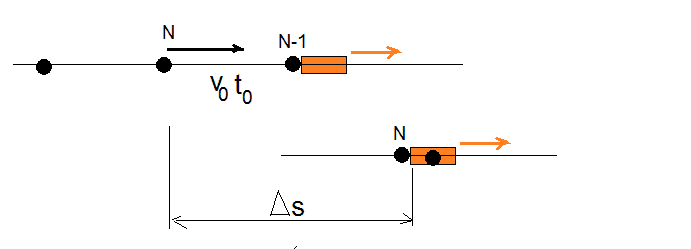Last edited:
•Biker
Thank you both, Ehild and the Biker...
Karol

6) How much time elapsed between the first bullet's hit and the Nth bullet's hit.
Did you solve this question? It seems like that it has a good concept inside it.

Did you solve this question? It seems like that it has a good concept inside it.
The time from the 1-st hit to the N-th hit is the sum of:
$$\sum^N_1 \frac{99+N}{100}t_0=\frac{t_0}{100}\left[ \sum^N_1 N+99N \right]=\frac{t_0}{100}\left[ \frac{N(1+N)}{2}+99N \right]=\frac{N(N+199)}{50}t_0$$

The time from the 1-st hit to the N-th hit is the sum of:
$$\sum^N_1 \frac{99+N}{100}t_0=\frac{t_0}{100}\left[ \sum^N_1 N+99N \right]=\frac{t_0}{100}\left[ \frac{N(1+N)}{2}+99N \right]=\frac{N(N+199)}{50}t_0$$
It seems that you have done something wrong,

I concluded another formula which passed few tests I gave it. You could do the same and see what your equation gives you but I am sure that something is wrong.

I will try to figure what might have gone wrong.

ehild
Homework Helper
It seems that you have done something wrong,

I concluded another formula which passed few tests I gave it. You could do the same and see what your equation gives you but I am sure that something is wrong.

I will try to figure what might have gone wrong.
First: you have to sum the intervalls from 2 to N.
And 2*100 in the denominator is not 50.

The sum should indeed be from 2 to N since, i think, for example if i have 3 bullets then i have to add 2 intervals:
$$\sum_2^N \frac{N+99}{100}=\sum_2^N N+(N-1)99=\frac{(N-1)(N+2)}{2}+99(N-1)=\frac{(n-1)(N+200)}{200}$$
But it's strange that if i take the sum from 1 to (N-1) it gives a different thing:
$$\sum_1^{N-1} \frac{N+99}{100}=\frac{N(N+197)}{200}$$
I thought only the number of members in the sum counts, but no.

The sum should indeed be from 2 to N since, i think, for example if i have 3 bullets then i have to add 2 intervals:
$$\sum_2^N \frac{N+99}{100}=\sum_2^N N+(N-1)99=\frac{(N-1)(N+2)}{2}+99(N-1)=\frac{(n-1)(N+200)}{200}$$
But it's strange that if i take the sum from 1 to (N-1) it gives a different thing:
$$\sum_1^{N-1} \frac{N+99}{100}=\frac{N(N+197)}{200}$$
I thought only the number of members in the sum counts, but no.
If you take the sum from 1 to N-1

with this function of impact time: ##F(N) = \frac{N+99}{100} t_o##
You are adding up the value ##t_o## into account which is not asked in the question, Also you are neglecting the impact time between the Nth bullet and N-1th

Thanks Biker

•Biker